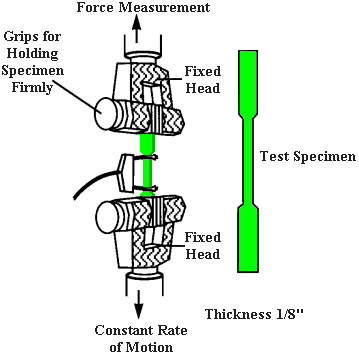Feb 26, 2016

Feb 26, 2016

Feb 26, 2016

# Tensile Strength or Tension Test

Tensile Test - Tensile Strength or Tension Test

## Definition:

Tensile strength of a material is the tension stress at which a material breaks or permanently deforms (changes shape)

sUTS = Pmax/Ao

There are three stages of Tensile Strength:

1. Yield Strength
2. Ultimate Strength and
3. Breaking strengthTensile strength of a material is the tension stress at which a material breaks or permanently deforms (changes shape) Toughness, Resilience, Poisson's ratio can also be found by the use of this testing technique. This data is plotted as load vs elongation and then converted to engineering stress (load/original area) vs engineering strain (fractional change in length over the test section assuming the deformation is uniform)

## Procedure of Strength Test:

A standard test piece (gauge length 8ft) is gripped at both ends in the jaws of UTM - Universal Testing Machine which slowly exerts an axial pull so that the steel is stretched until it breaks. The major parameters that describe the stress-strain curve obtained during the tension test are the:

1. Ultimate tensile strength (UTS)
2. Yield strength or yield point (sy)
3. Elastic modulus (E)
4. Percent elongation (?L%) and
5. The reduction in area (RA%).

Toughness, Resilience, Poisson's ratio can also be found by the use of this testing technique. This data is plotted as load vs elongation and then converted to engineering stress (load/original area) vs engineering strain (fractional change in length over the test section assuming the deformation is uniform.

### Engineering Stress:

Stress s = P / Ao ( Load/Initial cross-sectional area)

Strain = e = dl / lo (Elongation/Initial gauge length)

Engineering stress and strain are independent of the geometry of the specimen.

If the true cross section is measured during the test the True Stress and True Strain may be calculated. Tensile tests are applied on materials such as concrete, metals, plastics, wood, and ceramics etc.

## Units of Measurement:

Tensile testing systems use a number of different units of measurement. The International System of Units, or SI, recommends the use of either Pascals (Pa) or Newtons per square meter (N/m²) for describing tensile strength. In the United States, many engineers measure tensile strength in kilo-pound per square inch (KSI).